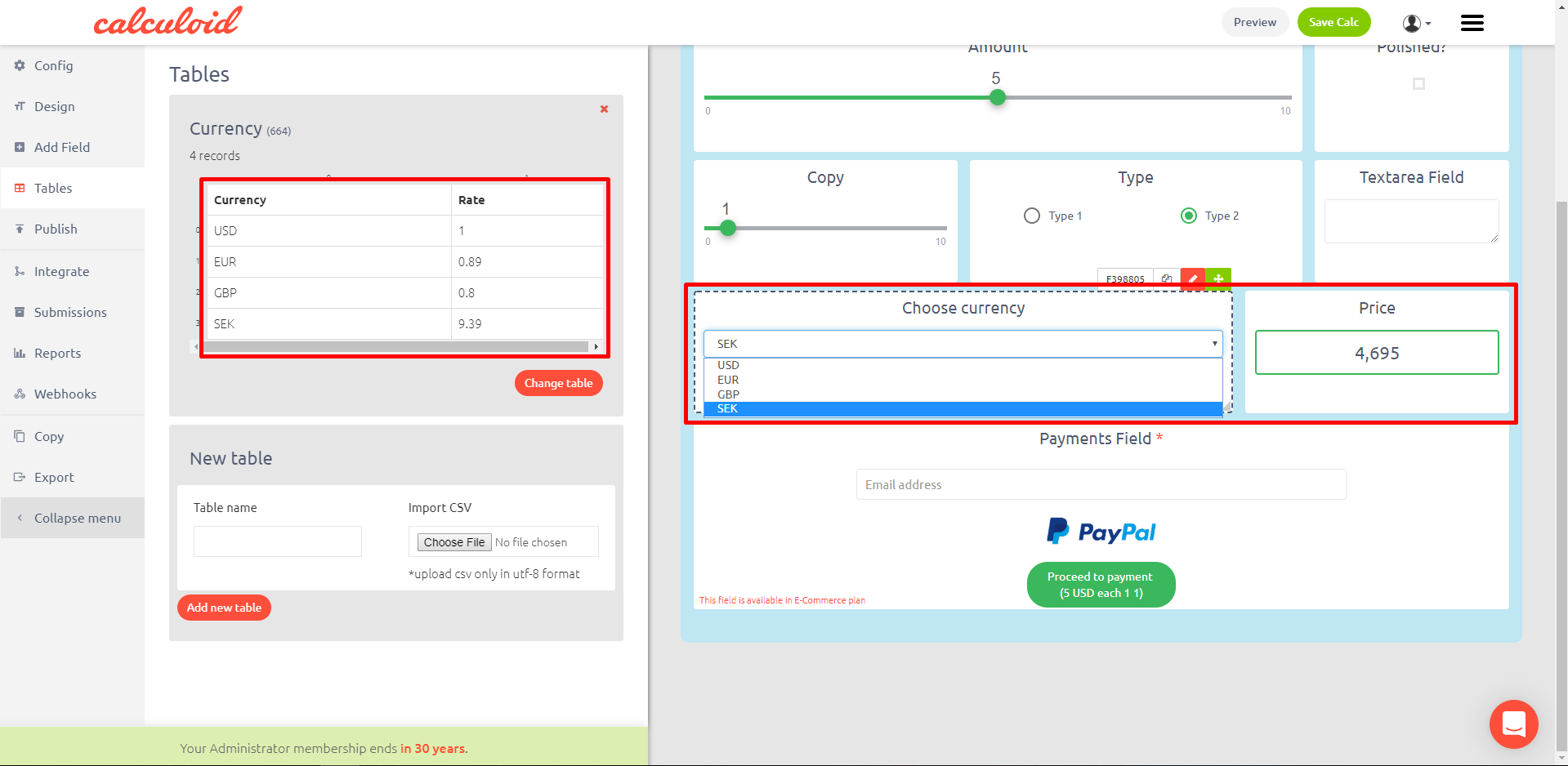Content

# Web calculators case studies #1 – CSV tables

CSV tables with functions in the formula field is a very strong feature which brings your calculator to a new level. Allows you to calculate based on back end static data, use discount codes for payments and even more.

Explore case studies and get inspired on how to use CSV tables to grow your business in Calculoid webinar recording.

It is very simple to create a CSV table with Excel or Google sheets. Please note that it should be CSV UTF-8 format and the size should be no more than 2 MB. To upload the table using 'Tables' on the left menu. Choose file in 'New table' section, name your table and click on 'Add new table'.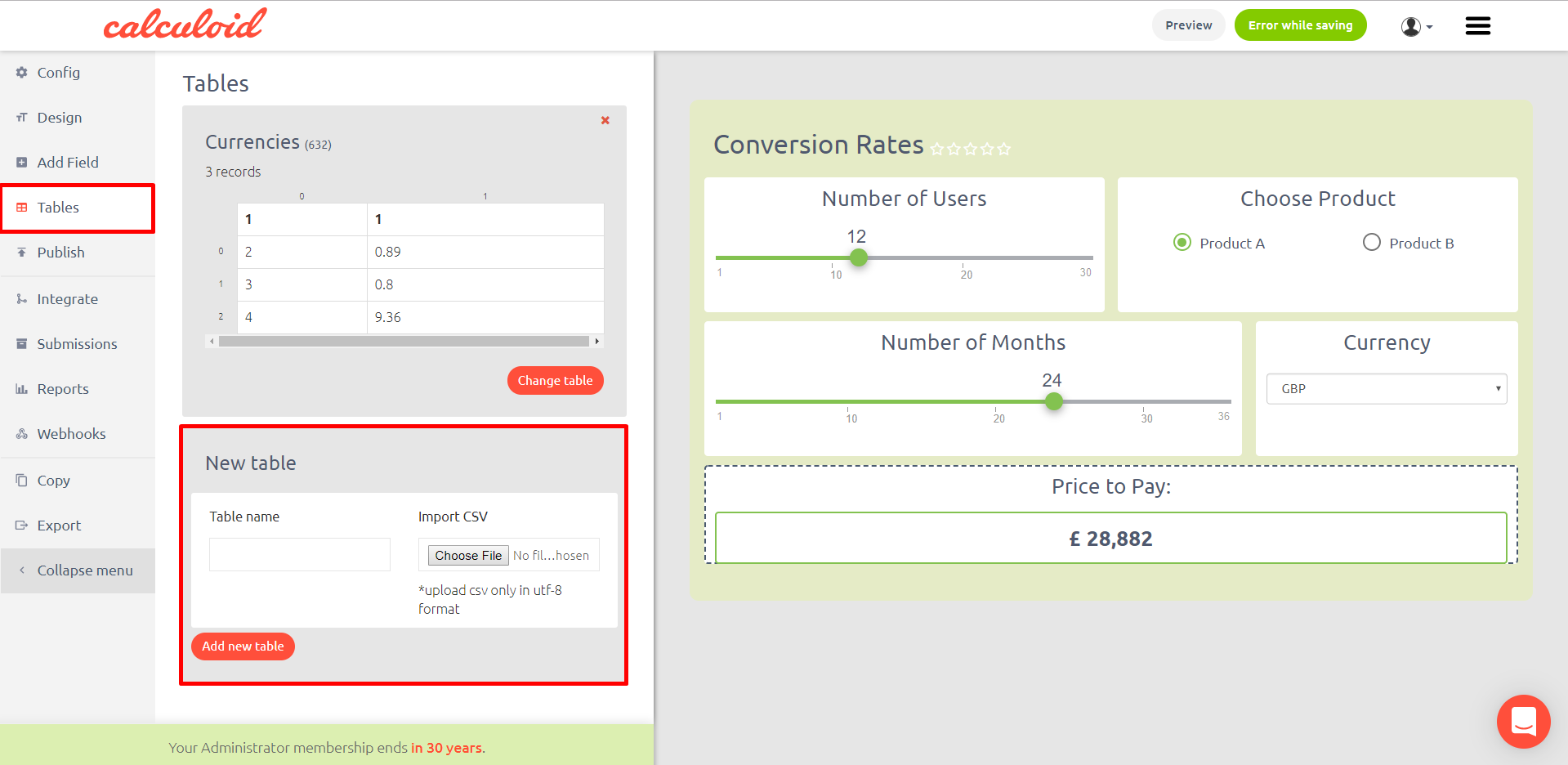## Case study 1 – How to prefill options in 'Select field'

There are two general ways of using a CSV table in Calculoid.

The first way is to use the file to prefill options in the 'Select field'. When the number of options is more than 10 it is much easier and faster to create a CSV and upload it to your calculator rather than typing in each option manually. To prefill options, once the table is uploaded, go to ‘Edit field’ > options > choose ‘table’ way of creating the options. Select the table needed and specify a column which will represent the ‘option name’ and a column for ‘option value’. It is that simple!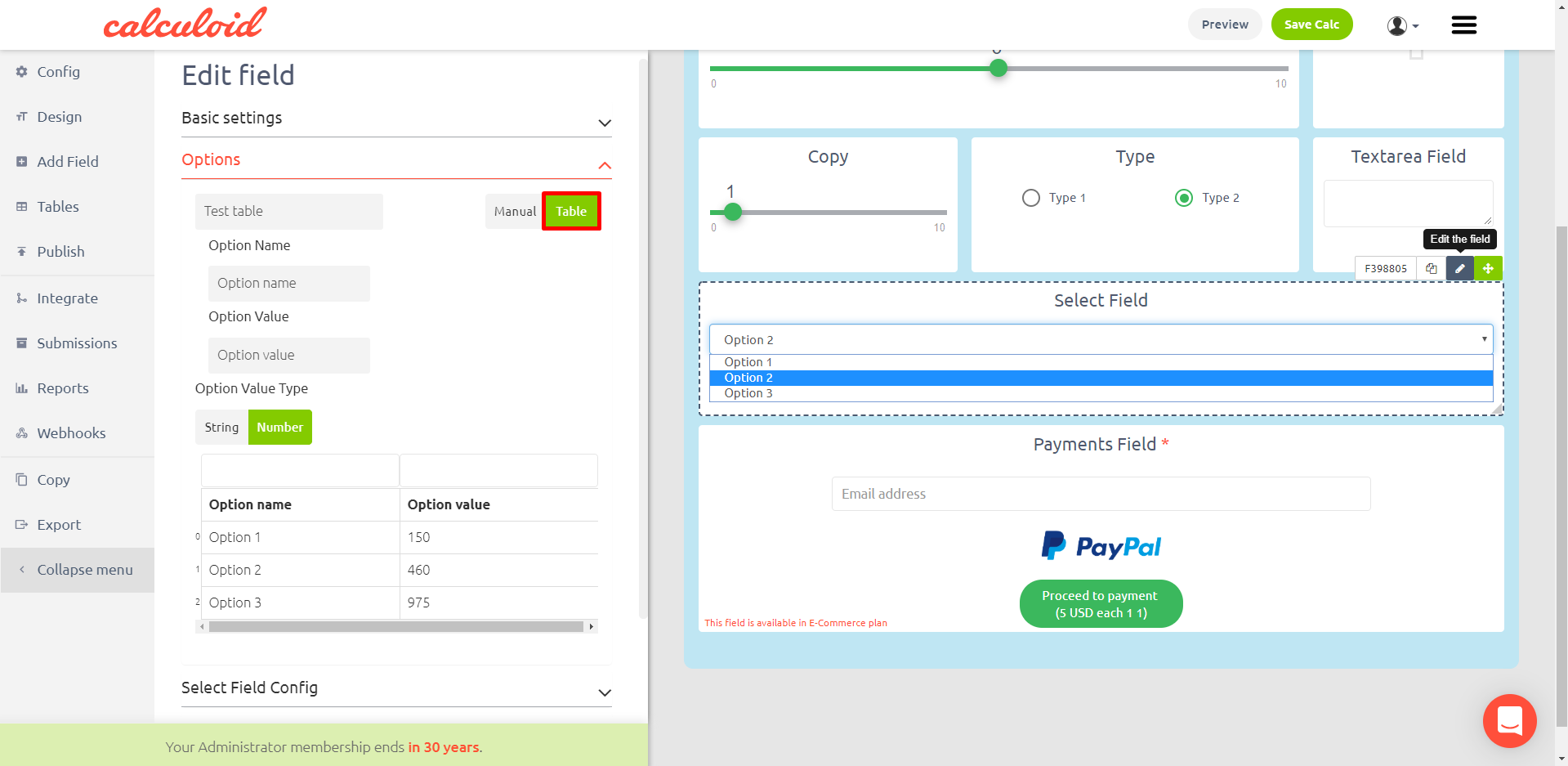The second way of implementing data from the CSV file requires a ‘formula field’ and a little bit of “magic” which is represented by lookup function.

Lookup function description: lookup (searchable value, searchable value column id, result column id, table id). All the IDs needed can be found in the ‘table’ tab in the left menu, above each table.

## Case study 2 – Discount codes

Imagine creating a pricing calculator. Discount code for your most wanted clients is something you wish to have in it. To add this function you need to create a CSV table where you put all the codes with the discount rates. Once you upload the table, add two fields: Text field (Discount code) and Formula field. Your clients type in the code they have and formula calculates the discount. Example of formula to calculate the discount: F397557 * F397560 * lookup (F397559, 0, 1, 603).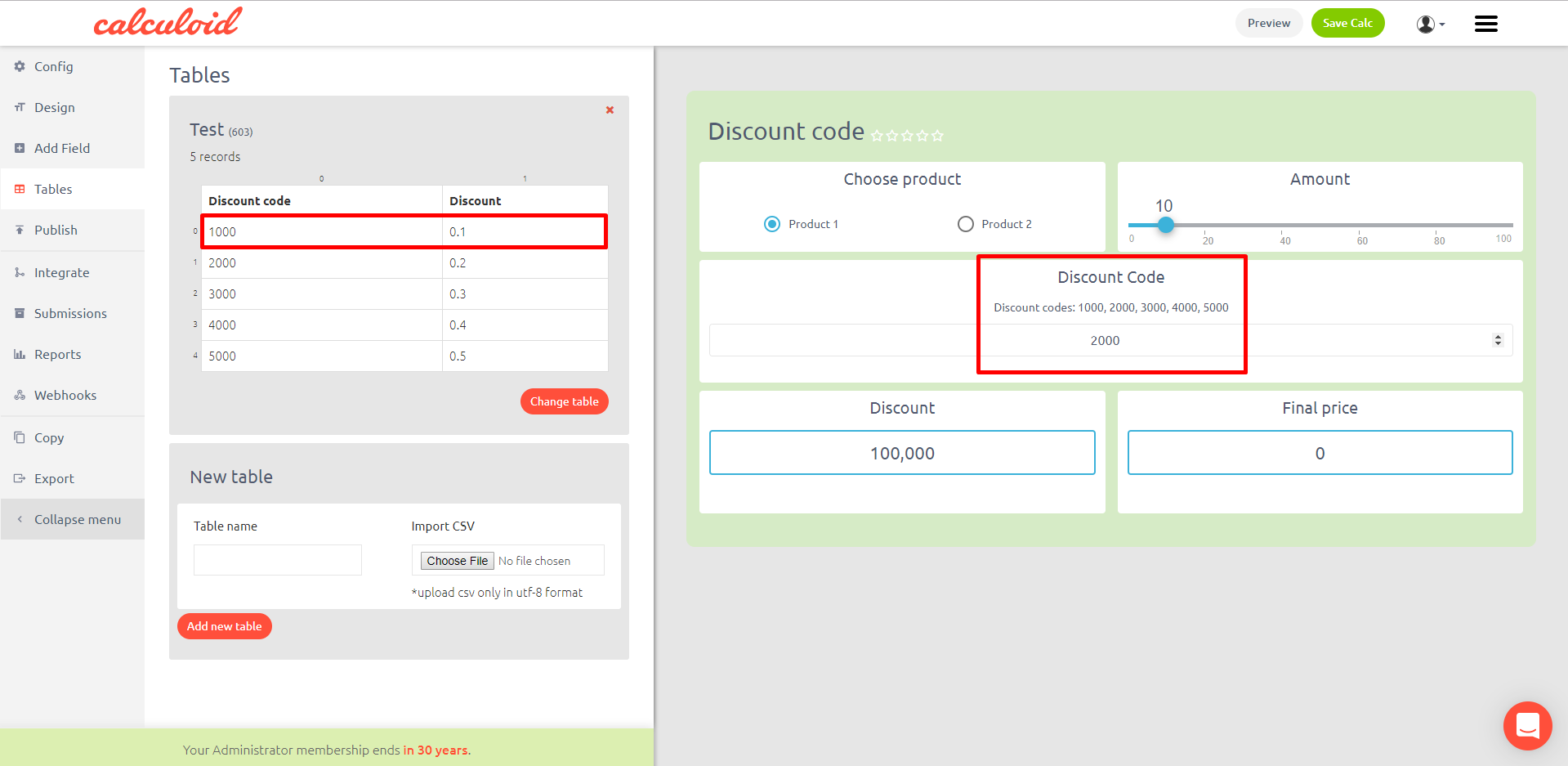Example of formula to calculate the discount: F397557 * F397560 * lookup (F397559, 0, 1, 603).

## Case study 3 – Text answer based on a code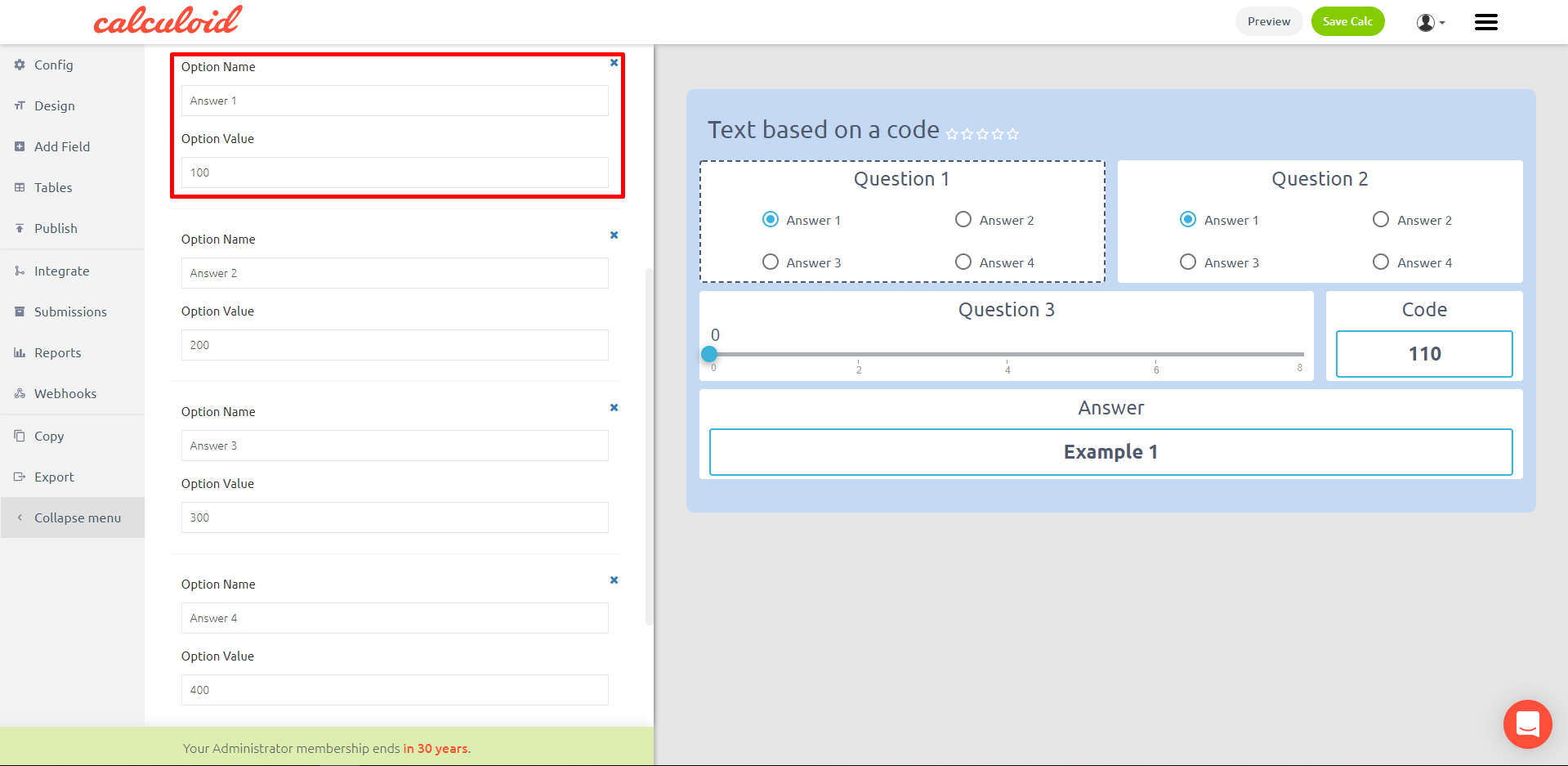## Case study 4 – Conversion rates

Many calculators require some static data on the back end. For example, you need to present your final price in different currencies. Store all the conversion rates in a CSV table and upload it. Then add this table to a ‘Select field’. In the formula multiply your final price by the dropdown with different currencies. Your results will be presented in the currency selected by your client.